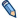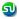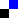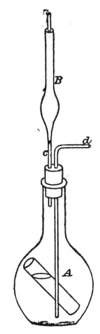# acidimetry## acidimetry defined in 1909 year

acidimetry - ACIDIMETRY;
acidimetry - See also alkalimetry. Acidimetry is the "measuring of acids," or determining the amount of free acid in an acidulous liquid. It does not indicate the nature of the acid, nor whether more than one acid is present. Three principal methods are available: (1) The strength of an acid solution may be approximately determined by its boiling-point; (2) by its specific gravity; (3) by the amount of carbonic acid gas evolved from bicarbonate of soda by a measured quantity of the acid liquid. This last is perhaps the simplest process, and that generally in use. The apparatus required is shown in Fig., and may be constructed by the operator. It consists of a wide-mouthed flask A, furnished with a tightly fitting cork, through which pass 2 glass tubes c d. The tube c terminates in a bulb B, filled with chloride of calcium; the bent tube d reaches nearly to the bottom of the flask. A carefully weighed quantity of pure bicarbonate of soda is introduced into the flask, and covered with distilled water. This done, a small glass test tube, containing a known volume of the acid to be examined (which must not be sufficient to decompose the whole of the alkali) is carefully lowered into the flask, in the position shown. The flask is then corked up, and accurately weighed on a delicate balance. After this, the acid in the test-tube is run out upon the alkali by causing the tube to slip into a horizontal position. By this means, a part of the alkali, equivalent to the amount of real acid in the liquid, is decomposed, the carbonic acid gas evolved escaping through the bulb-tube B; any moisture which may be carried upwards mechanically is absorbed by the chloride of calcium, whose affinity for water is well known. When the whole of the acid has been neutralised, and the disengagement of gas has ceased, air is sucked through the tube B in order to withdraw any gas remaining in the flask and tubes. When perfectly cool, the whole apparatus is re-weighed. The difference between the two weightings represents the weight of carbonic acid expelled, and from this the amount of real acid in the volume of liquid operated upon is calculated by multiplying it by the combining weight of the acid and dividing the product by 44, the combining weight of carbonic acid gas. Thus, suppose the weight of the apparatus before the experiment be 32.355 grm., and after the experiment 31.785 grm., the loss in weight, 0.570 grm., represents the amount of gas evolved from the bicarbonate of soda by the acid (say sulphuric acid). Then, 570x98/44 =1.27 grm. of real sulphuric acid, the amount contained in the volume of liquid taken for experiment. The same method applies to the estimation of any acid which decomposes carbonates, the combining weight of such acid being substituted for that of sulphuric acid used in the above example.

Another application of the same principle is a method devised by Fresenius and Will. The apparatus is shown in Fig., and consists of 2 small flasks, A B, A being slightly the larger. These are furnished with tightly-fitting corks, through each of which pass the glass tubes a b c, arranged as shown. The flask B is half filled with concentrated sulphuric acid, and in the other is placed the acid to be tested, accurately measured, and, if necessary, diluted with water. A test tube is now introduced into the flask A, in the same manner as described in the previous case; this tube contains bicarbonate of soda, in quantity more than sufficient to neutralise the whole of the acid contained in the sample. After carefully weighing the apparatus, the acid and alkali are allowed to mix; carbonic acid is evolved, passes through the sulphuric acid in the other flask, being thereby thoroughly dried, and escapes through the tube a. All effervescence having ceased, air is drawn through the 2 flasks by sucking at the extremity of the tube a, to remove any traces of carbonic acid remaining behind. When quite cool, the apparatus is re-weighed, the loss representing the amount of carbonic acid disengaged from the alkali. The calculation to find the total quantity of acid in the volume of liquid employed is, of course, the same as in the preceding example.

### Determining acidity of vinegar

This can be done by the neutralisation process, which is that of neutralising a certain quantity of vinegar with a normal solution of caustic soda. This solution contains 40 grm. of pure caustic soda in 1 litre, or 1 c.c. contains 0.04 grm. of the soda. The strength of this can be checked by titration with a normal solution of hydrochloric acid. To determine the acidity of vinegar, 20 c.c. are measured into a beaker. Into this are put a few drops of an alcoholic solution of the indicator phenolphthalein. The soda solution is added drop by drop (a burette being used), the vinegar being agitated all the time. Immediately there is the slightest excess of the soda a deep pink colour will be noticed, at which moment the addition of soda solution is stopped and the amount used is noted. The following calculation will then determine the strength of the vinegar; 1 c.c. of the soda solution (carrying 0.04 grm. of soda) is equal to 0.06 grm. of acetic acid, so that 21 c.c. of the former are needed to neutralise 20 c.c. of vinegar. The amount of acid in 100 pints of vinegar is 0.06 x 21 X 5 = 6.3 per cent.; as a rule the acetic acid in vinegar is 5 per cent, or a little less.

### Standard solutions, acid or alkali

These are solutions of exact known strengths. Usually they are" normal," but a deci-normal is also used; the latter being one tenth as strong as the former; of hydrochloric acid there are 36'5 grm. in one litre of the normal solution and one tenth of this amount in the deci-normal. To find the strength of an acid or an alkali, a certain quantity, say 20 c.c., is measured off, then it is titrated with either an alkali or acid, using an indicator, litmus for example, which changes colour as soon as the point of neutrality is arrived at. The standard solution is always added from a burette, drop by drop, and when neutralisation takes place, the amount of solution used is noted, and from this the amount of acid or alkali, present in the liquid being tested, is calculated.

## pictures for acidimetryacidometry, simple. >>>>acidometry, fresenius and will method. >>>>

## acidimetry defined in 1939 year

acidimetry - Acidimetry;
acidimetry - Chemical process of measuring the amount or strength of acid in solid or liquid substances. If the acid is known to be pure, its specific gravity may give sufficient indication of the strength of the acid, or this may be ascertained by the quantity of alkali needed exactly to neutralise or destroy the acidity. The process being reversible is also known as alkalimetry.

By taking a known amount of a pure alkali, such as sodium carbonate, and neutralising with it the acid of unknown strength, it is easy to calculate, from the chemical reaction, the amount of acid that was present. If on the other hand, a definite quantity of pure oxalic acid has been used to neutralise a solution of an alkali, the quantity of alkali present can be ascertained. It is necessary that the identity of the substance which is being tested should be established previously by chemical analysis. The test solutions used are called standard or normal solutions. They are prepared upon such a basis that they bear a relation to the molecular weight of the chemical employed. Only such substances are used for test solutions as can be obtained in a state of purity and remain unaltered in strength for a reasonable length of time.

As both the test solution and the substance that is being tested may be colourless, and afford no visible indication of the neutrality point, certain substances are employed which change colour according as the liquid is acid or alkaline. These are called indicators, among the best-known being litmusâ€”red with acids, blue with alkalis; cochineal, yellowish-red with acids, purple with alkalis: and phenol-phthalein, colourless with acids, red with alkalis. The choice of the indicator depends upon the nature of the test that is being made. If the acidimetry is conducted with solutions, and the relative volumes of the liquids furnish the data required, the method is called volumetric. Where the weight of the resulting reaction is the factor taken into account, the process is called gravimetric.

## near acidimetry in Knolik

 acid proof alloys homeletter "A"start from "AC" acidosis

definition of word "acidimetry" was readed 3139 times

Legal info